Homework Explained - Math Practice 101Dear guest, you are not a registered member. As a guest, you only have read-only access to our books, tests and other practice materials.

As a registered member you can:

Registration is free and doesn't require any type of payment information. Click here to Register.
Go to page:
Chapter 4: Nonproportional Relationships; Lesson 1:Representing Linear Nonproportional Relationships

Guided Practice

Make a table of values for each equation.

• Question 1

$$\text{y} = 2\text{x} + 5$$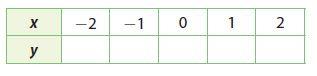• Type below:
• Question 2

$$\text{y} =\large\frac{3}{8}\small\text{x} - 5$$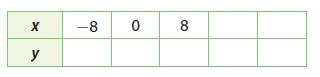• Type below:

Explain why each relationship is not proportional.

• Question 3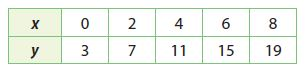First calculate $$\large\frac{y}{x}$$ for the values in the table.

• Question 4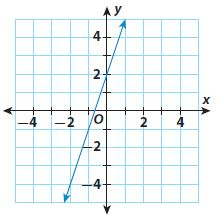Complete the table for the equation. Then use the table to graph the equation.

• Question 5

$$\text{y} = \text{x} - 1$$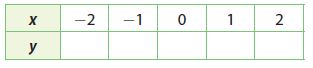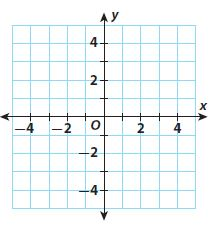• Type below:

Essential Question Check-In

• Question 6

How can you choose values for x when making a table of values representing a real world situation?

• Type below:

Yes, email page to my online tutor. (if you didn't add a tutor yet, you can add one here)